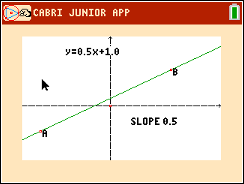# Activities

••• ##### Subject Area

• Math: Algebra I: Linear Functions

• ##### Author9-12

45 Minutes

• ##### Device
• TI-84 Plus
• TI-84 Plus Silver Edition
•TI-84 Plus C Silver Edition
•TI-84 Plus CE
• ##### Software

TI Connect™ CE

• ##### Accessories

TI Connectivity Cable

## Points, Lines and Slopes (Oh My!) - IB#### Activity Overview

In this activity students will explore the relationship between coordinates of points and locations on the coordinate plane, the relationships of lines with their equations, slopes and y-intercepts, and lastly, the slopes of parallel and perpendicular lines.

#### Objectives

• Students will graph linear functions and determine properties such as slope and y-intercept, describe how both change as the graph is rotated and translated, and understand that the coordinates of a line are the solution set to the equation of the line.
• Students will show that lines with equal slopes are parallel and slopes that are negative reciprocals are perpendicular.
•  Students will try to make a connection with how to understand these topics in IB Mathematics courses and on their final assessments

#### Vocabulary

• Parallel
• Perpendicular
• Reciprocal
• Slope